Ex 11.2

Chapter 11 Class 9 Constructions [Deleted]
Serial order wise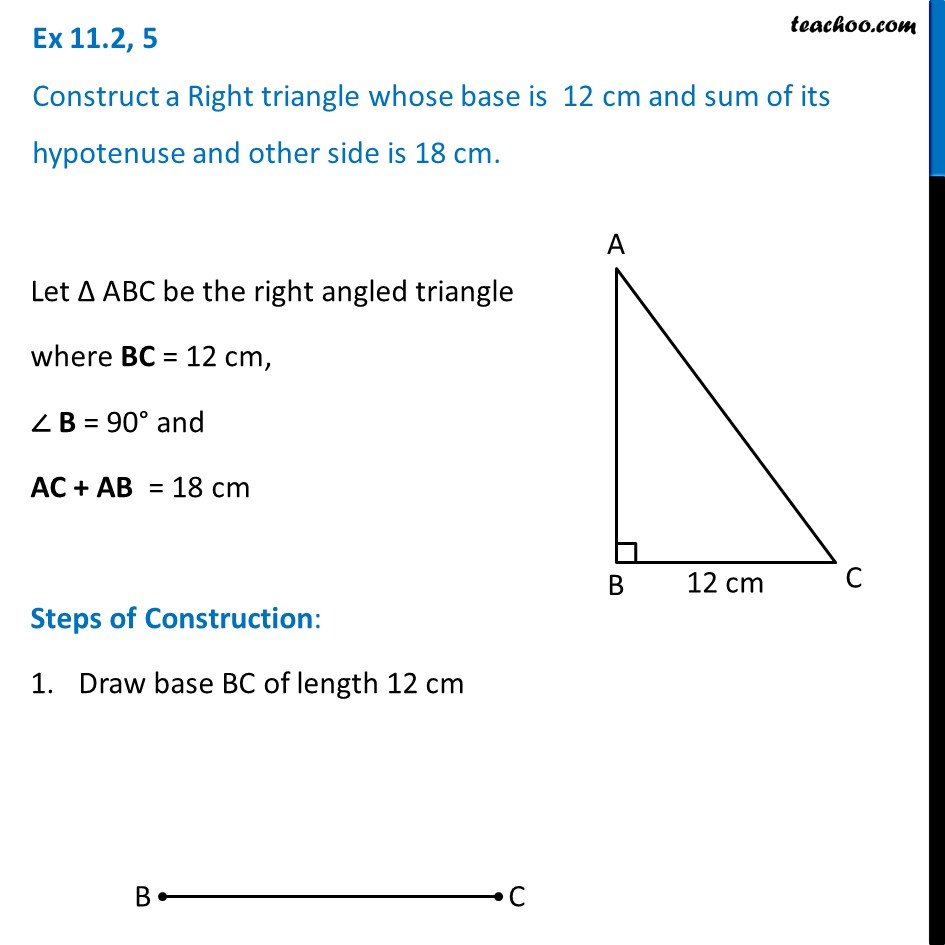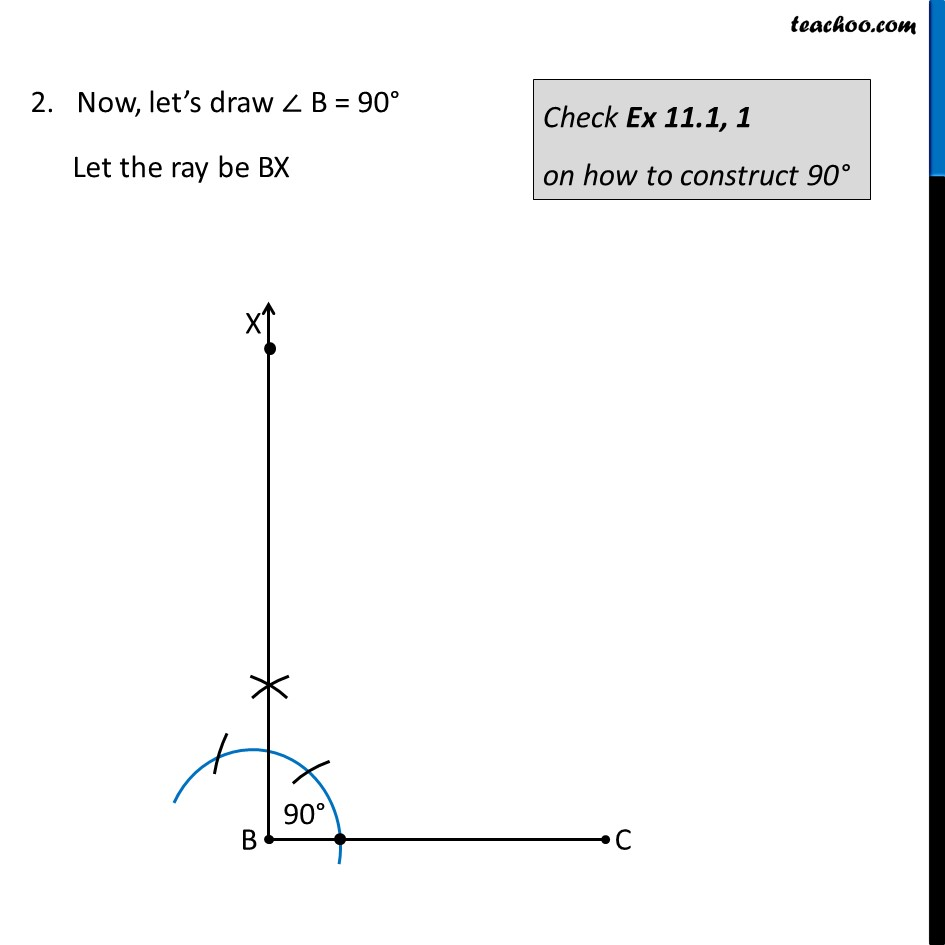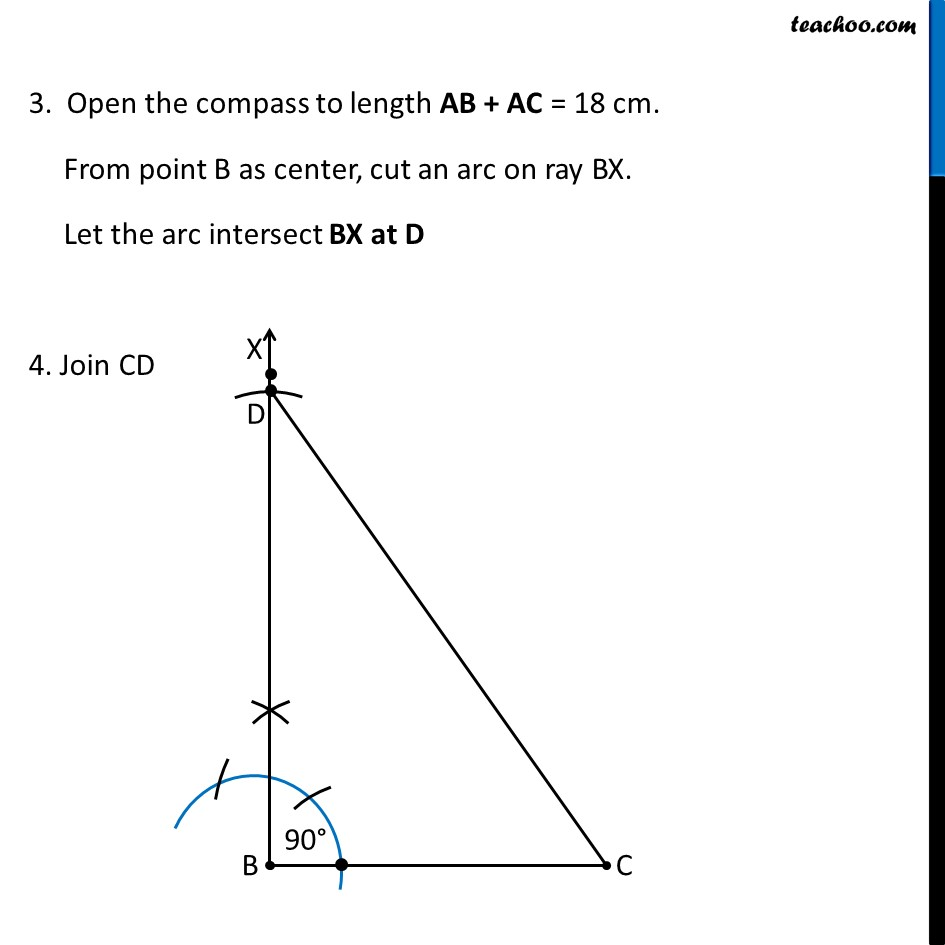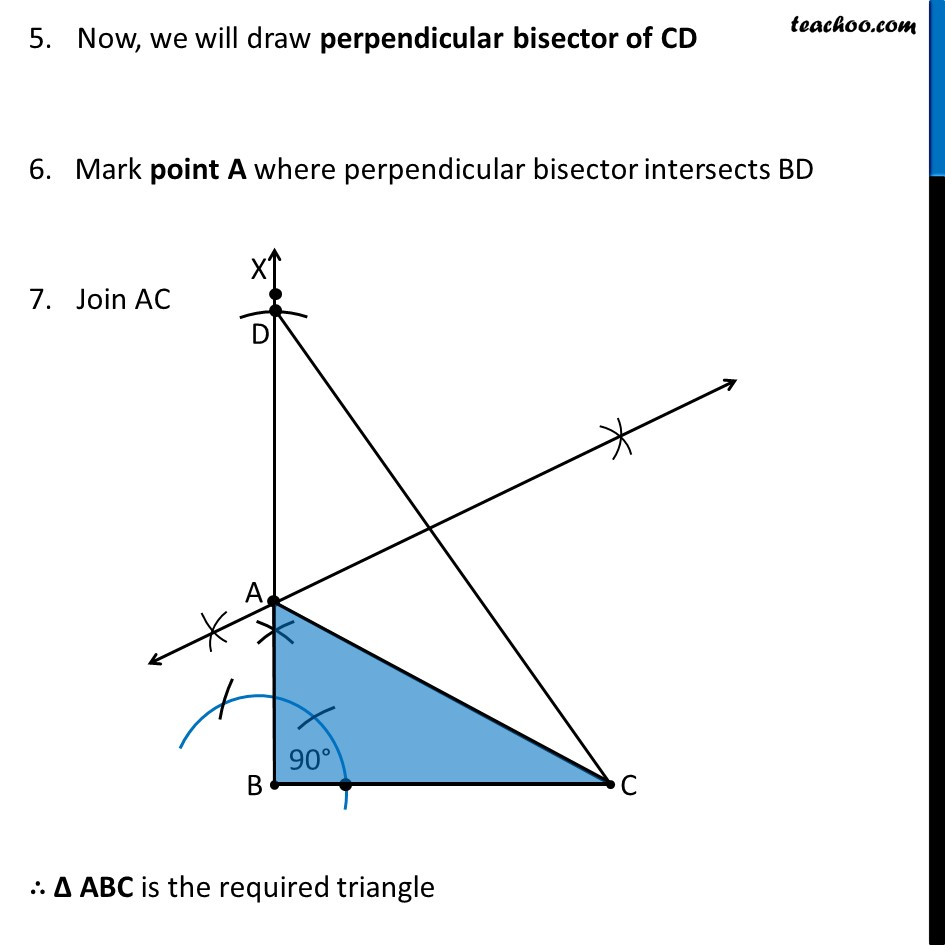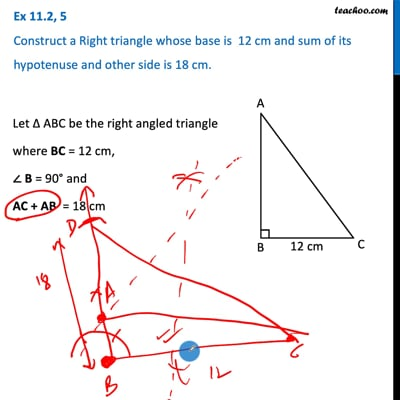This video is only available for Teachoo black users

Maths Crash Course - Live lectures + all videos + Real time Doubt solving!

### Transcript

Ex 11.2, 5 Construct a Right triangle whose base is 12 cm and sum of its hypotenuse and other side is 18 cm. Let Δ ABC be the right angled triangle where BC = 12 cm, ∠ B = 90° and AC + AB = 18 cm Steps of Construction: Draw base BC of length 12 cm 2. Now, let’s draw ∠ B = 90° Let the ray be BX Check Ex 11.1, 1 on how to construct 90° 3. Open the compass to length AB + AC = 18 cm. From point B as center, cut an arc on ray BX. Let the arc intersect BX at D 4. Join CD Now, we will draw perpendicular bisector of CD 6. Mark point A where perpendicular bisector intersects BD Join AC ∴ Δ ABC is the required triangle